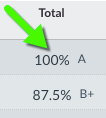A grade scheme is a way of organizing student performance on grade items into levels of achievement. Grade schemes can include any number of achievement levels. Each achievement level has its own range, and a grade (e.g., A, A-, B+) or performance indicator (e.g., Excellent, Good, Fair) to represent it. Once the scheme is applied, the achievement level appears after the numerical grade in the total column in the gradebook.All courses in Canvas have a default letter based grade scheme applied; however, you can create your own grade scheme.

### Default Grading Scheme in Canvas

A 94 to 100%
A- 90 to < 94%
B+ 87 to < 90%
B 83 to < 87%
B- 80 to < 83%
C+ 77 to < 80%
C 73 to < 77%
C- 70 to < 73%
D+ 67 to < 70%
D 63 to < 67%
D- 60 to < 63%
F 0 to < 60%

Note that the Registrar’s web grading system requires the use of letter grades. If you use a grading scheme that does not include letter grades, we encourage you to replace it with one that does.

## Grade Schemes and Assignments FAQ

Do you prefer to use letter grades when scoring assignments instead of point values? How do I add a grading scheme to an assignment explains how to do this. Here are some important FAQs to keep in mind when you choose to display letter grades for an assignment:

What happens if I enter a letter grade for a student's score instead of a point value?

If you’ve enabled a grading scheme for the assignment, Canvas will automatically convert the letter grade into a points-based score. For example, an “A-” might convert to a 9.3 out of 10. The converted score is based on the assignment’s grade scheme.

How can I change the "default percentage" that Canvas converts a letter grade to? (For example, when entering an "F", the score should be converted to 0)

Currently, it is not possible to change this without adjusting the range itself for the letter grade. When a letter grade is entered, Canvas converts it to a score by subtracting one percentage point from the value where the next highest letter grade’s range begins. For example, for a grade scheme where a “D-” begins at 60% (meaning anything below that is an “F”), then if you enter an “F” for a student’s score, Canvas will convert that to a 59%. If you wish to give a student a different score (such as 0%), you can simply enter the score instead of the letter grade.

What happens if I change my grade scheme after entering letter grades?

Student grades are always stored as point values, even if you set them to display as letter grades. Previously entered scores are not modified if you change your course or assignment grade scheme.

For example, say you have a grade scheme where the range for an “A-” is 90% to < 94%. Then you enter an “A-” for a student’s score on an assignment with this grade scheme enabled. Canvas will convert this to a 93% (see previous question). Later, if you change the grade scheme so that the range for an “A-” is 90% to < 93%, the student’s previously assigned score of 93% will not be modified, even though that score will now display as an “A” instead of an “A-”.

If you make changes to your grade schemes after you have already entered letter grades for an assignment, we recommend double-checking the assignment scores in SpeedGrader to ensure they still reflect the scores your students earned.